# How To Calculate Copper Cable Weight

By | December 19, 2022

As the demand for efficient electrical wiring continues to grow, so too does the need to understand how to calculate the weight of copper cables. Knowing the weight of your copper cable is essential for determining the size and length of cables you’ll need for any project. When it comes to understanding the physics behind calculating copper cable weight, it’s important to know the specific properties of the material, as well as understanding the different types available on the market.

When it comes to copper cable, there are a few different variables to consider when calculating the weight. From the specific type of copper cable being used, to the length and diameter, understanding all these factors can help you make an informed decision about your project. In this article, we’ll look at some of the basics of copper cable, as well as how to calculate the weight of copper cables. With some of these tips, you’ll be able to determine the correct weight for any copper wiring job you may have.

When looking at copper cables, it is important to understand that the weight will vary depending on the type of copper cable being used. Copper cabling comes in two main types: solid and stranded. Solid copper cables are composed of a single wire and are most commonly used for heavier-duty applications, such as those found in industrial settings. Stranded copper cables, on the other hand, are made up of several smaller wires braided together, and are most often used for lighter-duty applications, such as residential wiring.

The weight of any given copper cable is determined by the density of the material. The density of copper is 8.96 grams per cubic centimeter, so the weight of a given copper cable can be calculated by multiplying the volume of the cable by the density of the copper. To calculate the volume of a copper cable, you must first measure its length, width, and thickness. The volume of the cable is equal to the length multiplied by the width, and then multiplied by the thickness of the cable.

Once the volume of the cable has been determined, the weight can be calculated by multiplying the volume by the density of the copper. For example, if the length of a copper cable were two meters, the width were two millimeters, and the thickness were three millimeters, then the volume of the cable would be twelve cubic centimeters. Multiplying this volume by the density of copper, 8.96 grams per cubic centimeter, would give a total weight of 107.52 grams.

In addition to the volume of the cable, the weight of copper cables can also be affected by other factors, such as the number of strands in the cable, the type of insulation used, and the type of connectors attached to the cable. All of these factors should be taken into consideration when calculating the weight of a copper cable. For example, a cable with more strands and thicker insulation will require a greater amount of copper to produce the same amount of electricity than a cable with fewer strands and thinner insulation.

By understanding the different variables involved in calculating the weight of a copper cable, you can ensure that your wiring projects are as efficient and effective as possible. With some basic knowledge and understanding of the properties of copper, you can calculate the weight of any copper cable with ease.Fpv Wires And Connectors Sizes Cur Selection Oscar Liang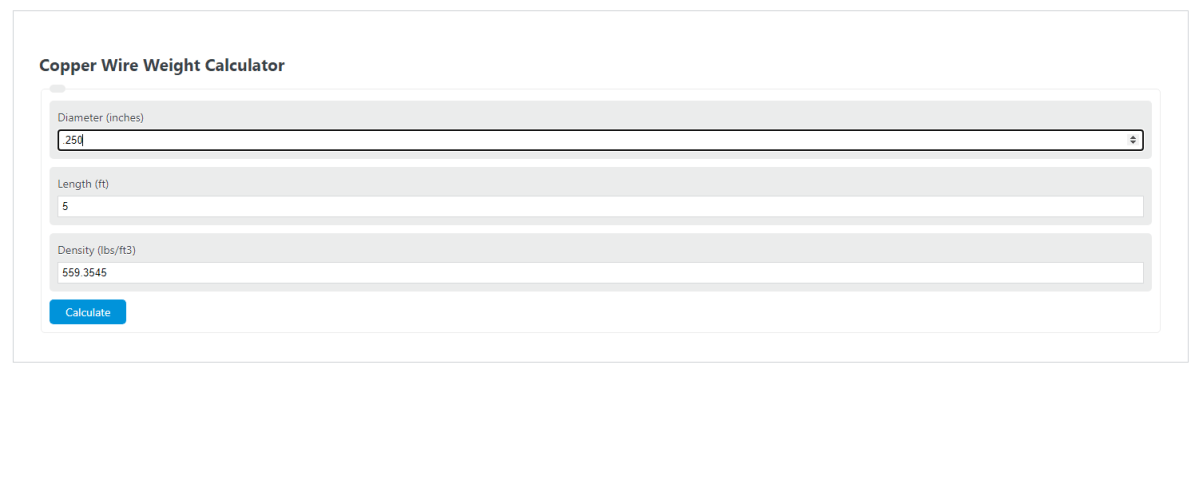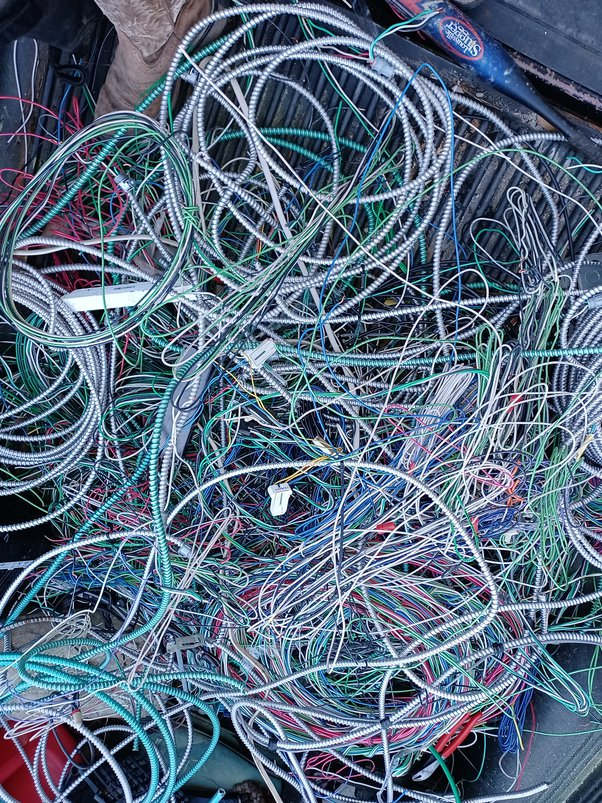How Much Does 100 Meters Of 0 5 Mm Diameter Copper Wire Weigh QuoraPower Cable Installation Guide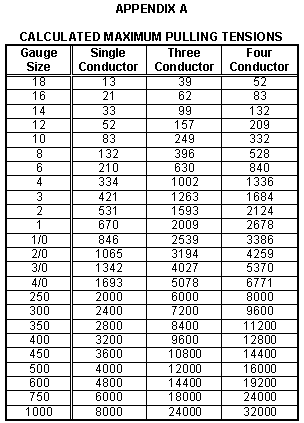Cable ManufacturerChart Of Awg Sizes In Metric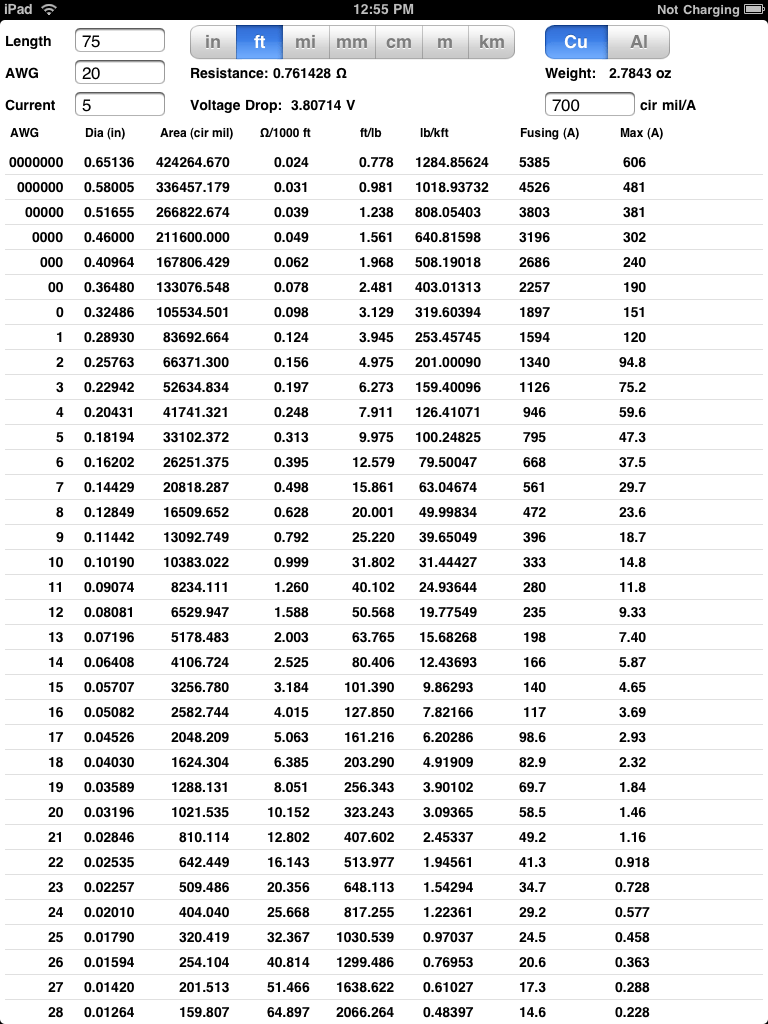Iphone And Ipad Software To Calculate Wire Resistance Voltage Drop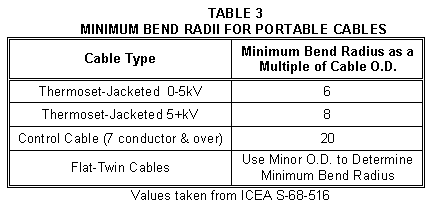Calculating Cable Pulling Tensions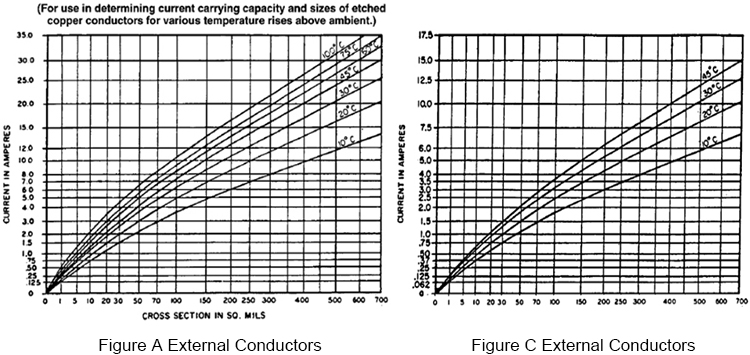Relationship Between Copper Weight Trace Width And Cur Carrying Capacity PcbcartHow To Calculate The Weight Of 150mm²tinned Copper Stranded WireCalculating Cable Pulling TensionsAluminium Copper Cur Carrying Capacity Calculation Chart In Sqmm Electrical4uHow Much Is Stripped Copper Wire Worth As S ToughnickelAluminum And Copper Wires Electrical Resistance Vs Cross Sectional Area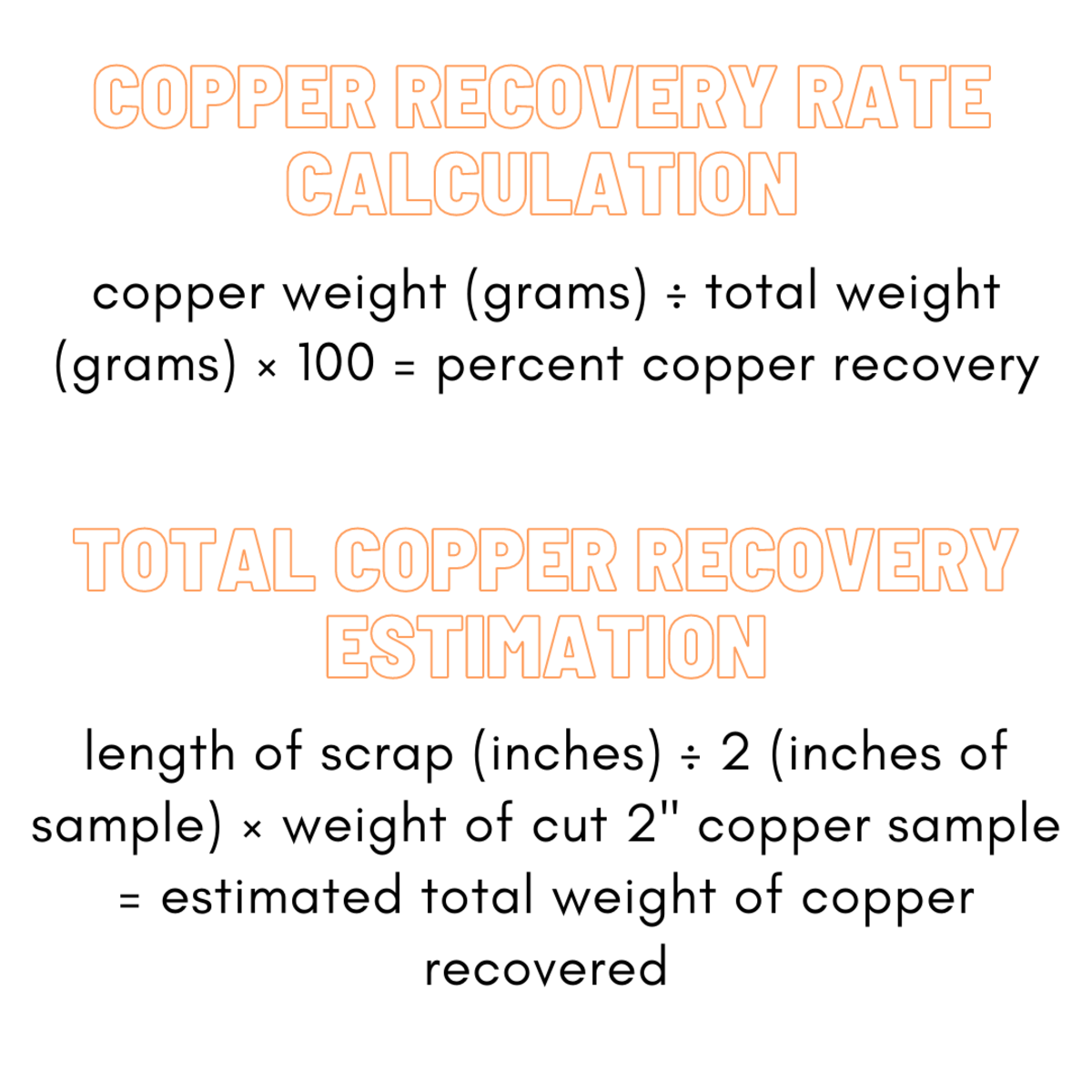How Much Is Stripped Copper Wire Worth As S Toughnickel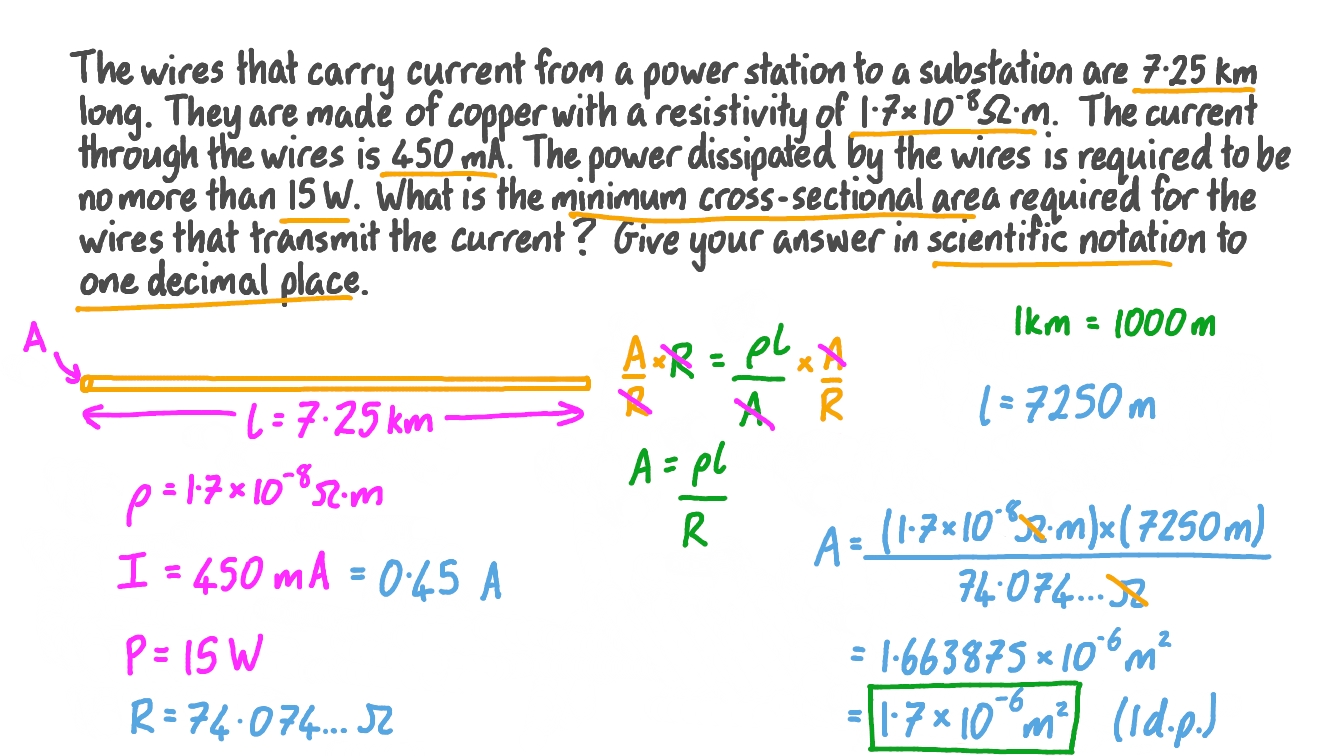Question Calculating The Minimum Cross Sectional Area Of A Wire NagwaWire Gauge CalculatorSteel Weight Calculator